# RD Sharma Class 10 Solutions Chapter 7 Triangles Ex 7.7

## RD Sharma Class 10 Solutions Chapter 7 Triangles Ex 7.7

These Solutions are part of RD Sharma Class 10 Solutions. Here we have given RD Sharma Class 10 Solutions Chapter 7 Triangles Ex 7.7

Other Exercises

Question 1.
If the sides of a triangle are 3 cm, 4 cm and 6 cm long, determine whether the triangle is a right-angled triangle. (C.B.S.E. 1992)
Solution:
We know that if the square of the hypotenuse (longest side) is equal to the sum of squares of other two sides then it is right triangle
Now the sides of a triangle are 3 cm, 4 cm and 6 cm
(Longest side)² = (6)² = 36
and sum of two smaller sides = (3)² + (4)² = 9 + 16 = 25
36 ≠ 25
It is not a right-angled triangle

Question 2.
The sides of certain triangles are given below. Determine which of them are right triangles :
(i) a = 1 cm, b = 24 cm and c = 25 cm
(ii) a = 9 cm, b = 16 cm and c = 18 cm
(iii) a = 1.6 cm, b = 3.8 cm and c = 4 cm
(iv) a = 8 cm, b = 10 cm and c = 6 cm (C.B.S.E. 1992)
Solution:
We know that if the square of hypotenuse is equal to the sum of squares of other two sides, then it is a right triangle
(i) Sides of a triangle are a = 7 cm, b = 5.24 cm and c = 25 cm
(Longest side)² = (25)² = 625
Sum of square of shorter sides = (7)² + (24)² = 49 + 576 = 625
625 = 625
This is right triangle
(ii) Sides of the triangle are a = 9 cm, b = 16 cm, c = 18 cm
(Longest side)² = (18)² = 324
and sum of squares of shorter sides = (9)² + (16)² = 81 + 256 = 337
324 ≠ 337
It is not a right-angled triangle
(iii) Sides of the triangle are a = 1.6 cm, 6 = 3.8 cm, c = 4 cm
(Longest side)² = (4)² =16
Sum of squares of shorter two sides + (1.6)² + (3.8)² = 2.56 + 14.44 = 17.00
16 ≠ 17
It is not a right triangle
(iv) Sides of the triangle are a = 8 cm, b = 10 cm, c = 6 cm
(Longest side)² = (10)² = 100
Sum of squares of shorter sides = (8)² + (6)² = 64 + 36 = 100
100 = 100
It is a right triangle

Question 3.
A man goes 15 metres due west and then 8 metres due north. How far is he from the starting point ?
Solution:
Let a man starts from O, the starting point to west 15 m at A and then from A, 8 m due north at B
Join OB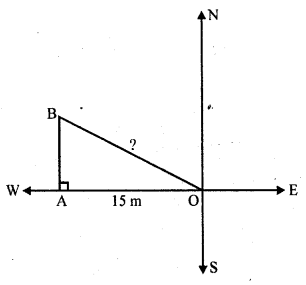Now in right ∆OAB
OB² = OA² + AB² (Pythagoras Theorem)
OB² = (15)² + (8)² = 225 + 64 = 289 = (17)²
OB = 17
The man is 17 m away from the starting point

Question 4.
A ladder 17 m long reaches a window of a building 15 m above the ground. Find the distance of the foot of the ladder from the building.
Solution:
Length of ladder = 17 m
Height of window = 15 m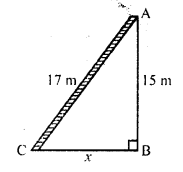Let the distance of the foot of ladder from the building = x
Using Pythagoras Theorem
AC² = AB² + BC²
=> (17)² = (15)² + x²
=> 289 = 225 + x²
=> x² = 289 – 225
=> x² = 64 = (8)²
x = 8
Distance of the foot of the ladder from the building = 8m

Question 5.
Two poles of heights 6 m and 11 m stand on a plane ground. If the distance between their feet is 12 m, find the distance between their tops. (C.B.S.E. 1996C, 2002C)
Solution:
Two poles AB and CD which are 6 m and 11 m long respectively are standing oh the ground 12 m apart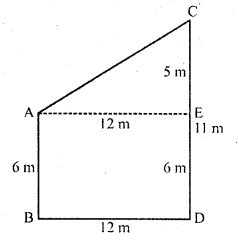Draw AE || BD so that AE = BD = 12 m and ED = AB = 6 m
Then CE = CD – ED = 11 – 6 = 5 m
Now in right ∆ACE
Using Pythagoras Theorem,
AC² = AE² + EC² = (12)² + (5)² = 144 + 25 = 169 = (13)²
AC = 13
Distance between their tops = 13 m

Question 6.
In an isosceles triangle ABC, AB = AC = 25 cm, BC = 14 cm. Calculate the altitude from A on BC. (C.B.S.E. 1994)
Solution:
∆ABC is an isosceles triangle in which AB = AC = 25 cm .
AD ⊥ BC BC = 14 cmPerpendicular AD bisects the base i.e . BD = DC = 7 cm
In right ∆ABD,
AB² = AD² + BD² (Pythagoras Theorem)
=> (25)² = AD² + (7)²
=> 625 = AD² + 49
=> AD² = 625 – 49
=> AD² = 576 = (24)²

Question 7.
The foot of a ladder is 6 m away from a wall and its top reaches a window 8 m above the ground. If the ladder is shifted in such a way that its foot is 8 m away from the wall, to what height does its tip reach ?
Solution:
In first case,
The foot of the ladder are 6 m away from the wall and its top reaches window 8 m high
Let AC be ladder and BC = 6 m, AB = 8 m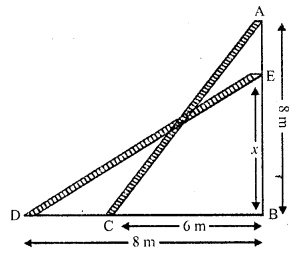Now in right ∆ABC,
Using Pythagoras Theorem
AC² = BC² + AB² = (6)² + (8)² = 36 + 64 = 100 = (10)²
AC = 10 m
In second case,
ED = AC = 10 m
BD = 8 m, let ED = x
ED² = BD² + EB²
=> (10)² = (8)² + x²
=> 100 = 64 + x²
=> x² = 100 – 64 = 36 = (6)²
x = 6
Height of the ladder on the wall = 6 m

Question 8.
Two poles of height 9 m and 14 m stand on a plane ground. If the distance between their feet is 12 m, find the distance between their tops.
Solution:
Let CD and AB be two poles which are 12 m apart
AB = 14 m, CD = 9 m and BD = 12 m
From C, draw CE || DB
CB = DB = 12 m
EB = CD = 9 m
and AE = 14 – 9 = 5 m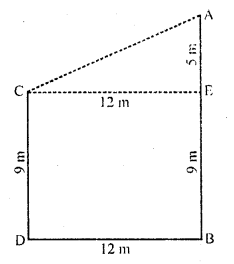Now in right ∆ACE,
AC² = AE² + CE² (Pythagoras Theorem)
= (5)² + (12)²
= 25 + 144 = 169 = (13)²
AC = 13
Distance between their tops = 13 m

Question 9.
Using Pythagoras theorem, determine the length of AD in terms of b and c shown in the figure. (C.B.S.E. 1997C)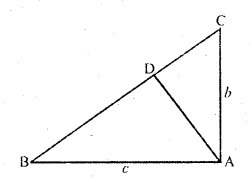Solution:
In right ∆ABC, ∠A = 90°
AB = c, AC = b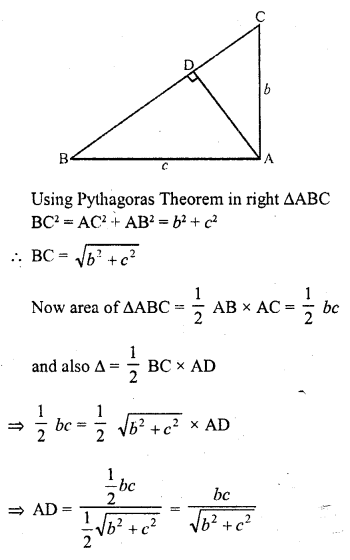Question 10.
A triangle has sides 5 cm, 12 cm and 13 cm. Find the length to one decimal place, of the perpendicular from the opposite vertex to the side whose length is 13 cm. (C.B.S.E. 1992C)
Solution:
A triangle has sides 5 cm, 12 cm and 13 cm
(Longest side)² = (13)² = 169
Sum of squares of shorter sides = (5)² + (12)² = 25 + 144= 169
169 = 169
It is a right triangle whose hypotenuse is 13 cm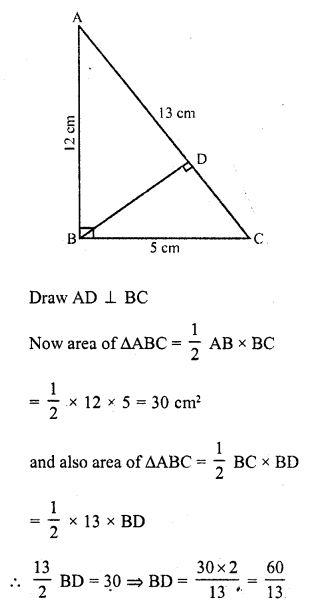BD = 4.6 cm

Question 11.
ABCD is a square, F is the mid point of AB. BE is one third of BC. If the area of ∆FBE = 108 cm² find the length of AC. (C.B.S.E. 1995)
Solution:
In square ABCD, F is mid point of AB i.e.,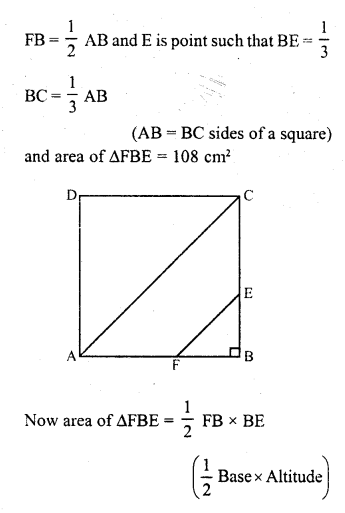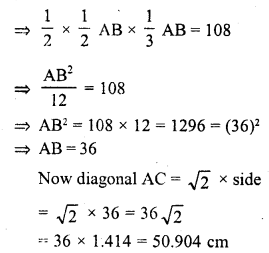Question 12.
In an isosceles triangle ABC, if AB = AC = 13 cm and the altitude from A on BC is 5 cm, find BC. (C.B.S.E. 2000)
Solution:
In ∆ABC, AB = AC
AB = AC = 13 cm, AD = 5 cm
BD = $$\frac { 1 }{ 2 }$$ BC
=> BC = 2BD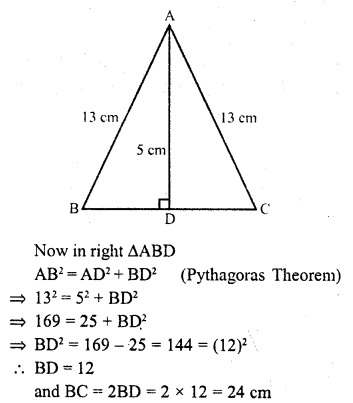Question 13.
In a ∆ABC, AB = BC = CA = 2a and AD ⊥ BC. Prove that
(ii) area (∆ABC) = √3 a² (C.B.S.E. 1991)
Solution:
In ∆ABC, AB = BC = AC = 2a
BD = DC = $$\frac { 1 }{ 2 }$$ BC = a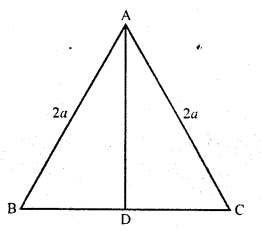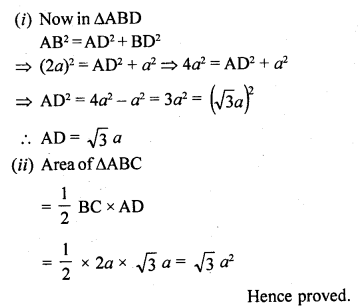Question 14.
The lengths of the diagonals of a rhombus are 24 cm and 10 cm. Find each side of the rhombus. (C.B.S.E. 1993C)
Solution:
ABCD is a rhombus whose diagonals AC = 24 cm and BD = 10 cm
The diagonals of a rhombus bisect eachother at right angles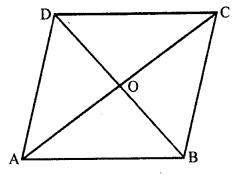AO = OC = $$\frac { 24 }{ 2 }$$ = 12 cm
and BO = OD = $$\frac { 10 }{ 2 }$$ = 5 cm
Now in right ∆AOB,
AB² = AO² + BO² (Pythagoras Theorem)
(12)² + (5)² = 144 + 25 = 169 = (13)²
AB = 13
Each side of rhombus = 13 cm

Question 15.
Each side of a rhombus is 10 cm. If one of its diagonals is 16 cm, find the length of the other diagonal.
Solution:
In rhombus ABCD, diagonals AC and BD bisect eachother at O at right angles
Each side = 10 cm and one diagonal AC = 16 cm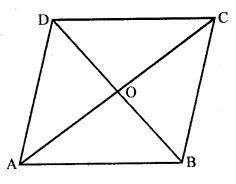AO = OC = $$\frac { 16 }{ 2 }$$ = 8 cm
Now in right angled triangle AOB,
AB² = AO² + OB² (Pythagoras Theorem)
(10)² = (8)² + (BO)²
=> 100 = 64 + BO²
=> BO² = 100 – 64 = 36 = (6)²
BO = 6
BD = 2BO = 2 x 6 = 12 cm

Question 16.
Calculate the height of an equilateral triangle each of whose sides measures 12 cm.
Solution:
Each side of the equilateral ∆ABC = 12 cm
AD ⊥ BC which bisects BC at D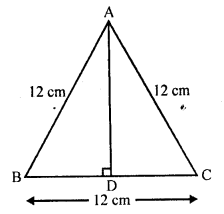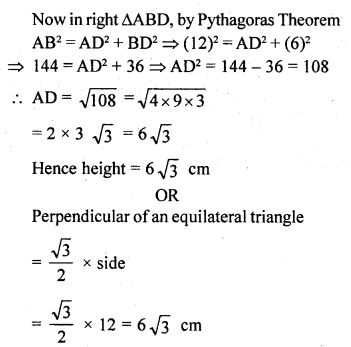BD = DC = $$\frac { 12 }{ 2 }$$ = 6 cm

Question 17.
In the figure, ∠B < 90° and segment AD ⊥ BC. Show that:
(i) b² = h² + a² + x² – 2ax
(ii) b² = a² + c² – 2ax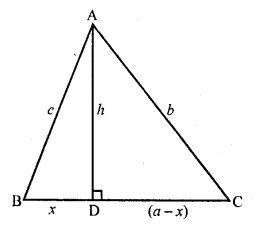Solution:
Given : In ∆ABC, ∠B < 90°
AD = c, BC = a, CA = b AD = h, BD = x, DC = a – x
To prove: (i) b² = h² + a² + x² – 2ax
(ii) b² = a² + c² – 2ax
Proof: (i) In right ∆ADC, AC² = AD² + DC² (Pythagoras Theorem)
=> b² = h² + (a – x)² = h² + a² + x² – 2ax
c² = h² + x² ….(i)
b² = h² + a² + x² – 2ax = h² + x² + a² – 2ax
= c² + a² – 2ax {From (i)}
= a² + c² – 2ax
Hence proved.

Question 18.
In an equilateral ∆ABC, AD ⊥ BC, prove that AD² = 3 BD². (C.B.S.E. 2002C)
Solution:
Given : ∆ABC is an equilateral in which AB = BC = CA .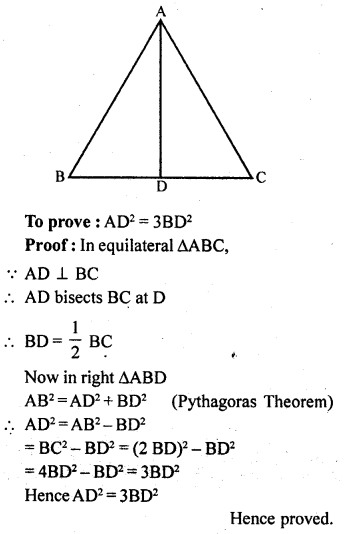Question 19.
∆ABD is a right triangle right-angled at A and AC ⊥ BD. Show that
(i) AB² = BC.BD
(ii) AC² = BC.DC
(iv) $$\frac { { AB }^{ 2 } }{ { AC }^{ 2 } }$$ = $$\frac { BD }{ DC }$$ [NCERT]
Solution:
In ∆ABD, ∠A = 90°
AC ⊥ BD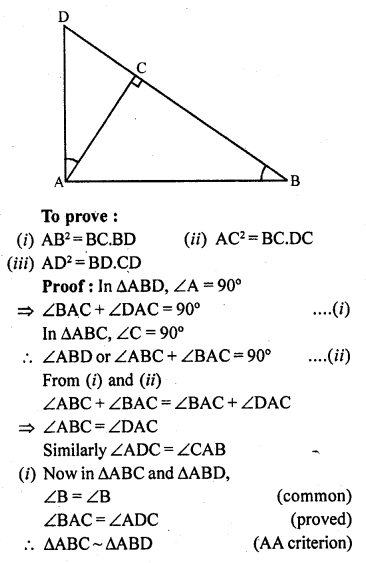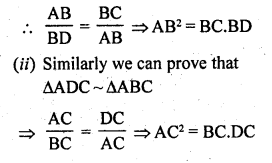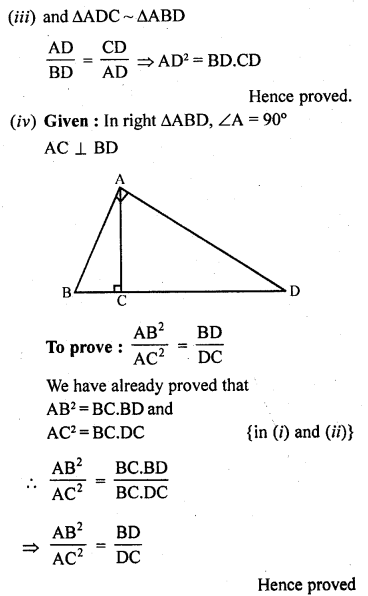Question 20.
A guy wire attached to a vertical pole of height 18 m is 24 m long and has a stake attached to the other end. How far from the base of the pole should the stake be driven so that the wire will be taut ?
Solution:
Let AB be the vertical pole whose height = 18 m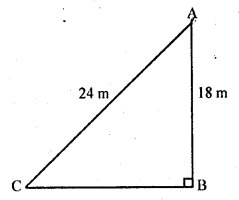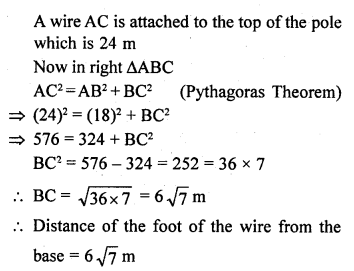Question 21.
Determine whether the triangle having sides (a – 1) cm, 2 √a cm and (a + 1) cm is a right angled triangle. [CBSE 2010]
Solution:
Sides of a triangle are (a – 1) cm, 2 √a cm and (a + 1) cm
Let AB = (a – 1) cm BC = (a + 1) cm
and AC = 2 √a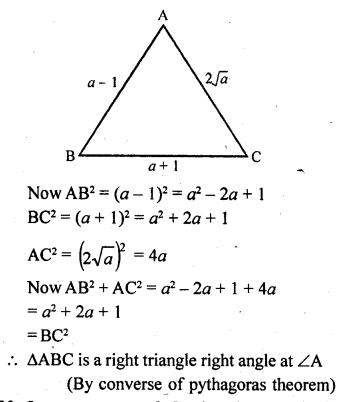Question 22.
In an acute-angled triangle, express a median in terms of its sides.
Solution:
In acute angled ∆ABC,
AD is median and AL ⊥ BC
Using result of theorem, sum of the squares of any two sides is equal to the twice the square of half of the third side together with twice the square of the median which bisects the third side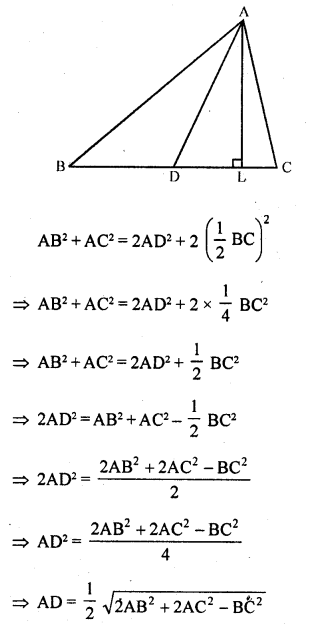Question 23.
In right-angled triangle ABC in which ∠C = 90°, if D is the mid point of BC, prove that AB² = 4AD² – 3AC².
Solution:
Given : In right ∆ABC, ∠C = 90°
D is mid point of BC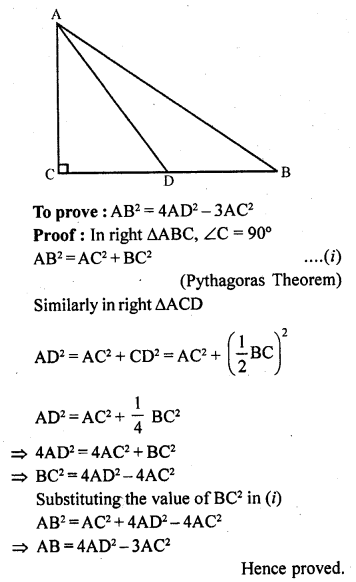Question 24.
In the figure, D is the mid-point of side BC and AE ⊥ BC. If BC = a, AC = b, AB = c, ED = x, AD = p and AE = h, prove that :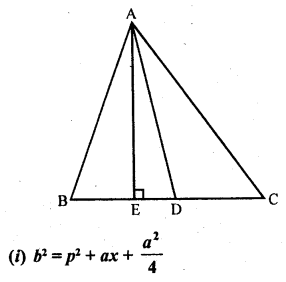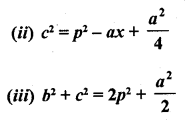Solution: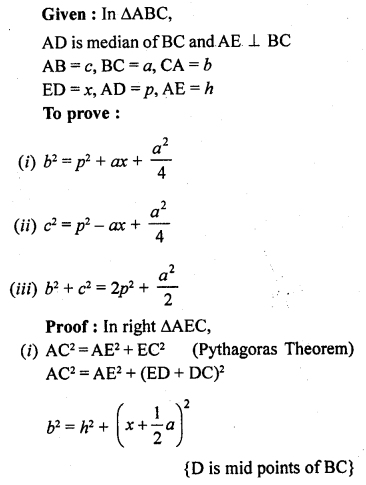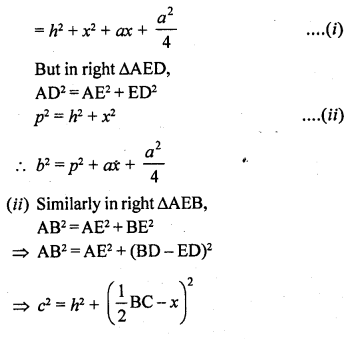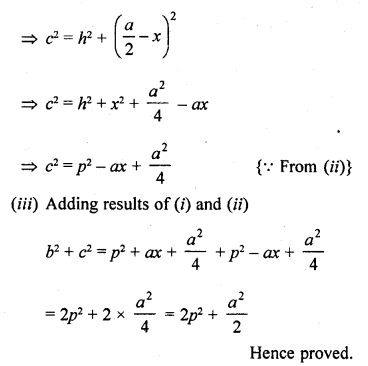Question 25.
In ∆ABC, ∠A is obtuse, PB x AC and QC x AB. Prove that:
(i) AB x AQ = AC x AP
(ii) BC² = (AC x CP + AB x BQ)
Solution:
Given : In ∆ABC, ∠A is an obtuse angle PB x AC and QC x AB on producing them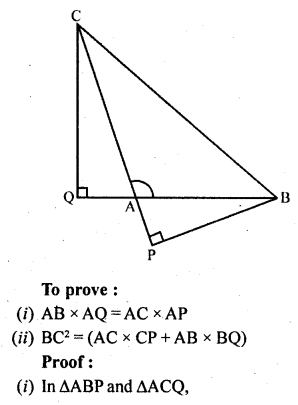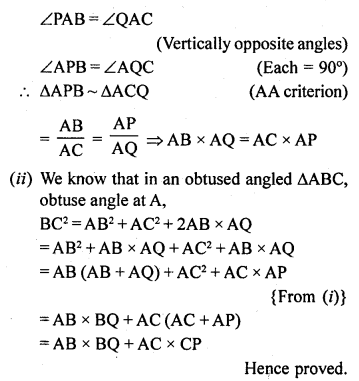Question 26.
In a right ∆ABC right-angled at C, if D is the mid-point of BC, prove that BC² = 4 (AD² – AC²).
Solution:
Given : In ∆ABC, ∠C = 90°
D is mid-point of BC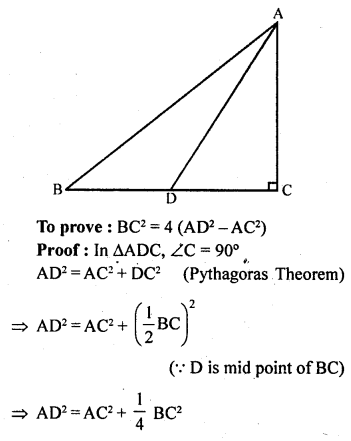=> 4AD² = 4AC² + BC²
=> BC² = 4AD² – 4AC²
=> BC² = 4 (AD² – AC²)
Hence proved.

Question 27.
In a quadrilateral ABCD, ∠B = 90°, AD² = AB² + BC² + CD², prove that ∠ACD = 90°.
Solution:
Given : ABCD is a quadrilateral in which ∠B = 90° and AD² = AB² + BC² + CD²
To prove : ∠ACD = 90°
Construction : Join AC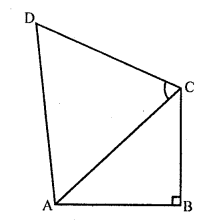proof : In right ∆ABC, ∠B = 90°
AC² = AB² + BC² ….(i) (Pythagoras Theorem)
But AD² = AB² + BC² + CD² (given)
=> AD² = AC² + CD² {From (i)}
∆ACD is a right angle with right angle ACD
Hence proved.

Question 28.
An aeroplane leaves an airport and flies due north at a speed of 1000 km/hr. At the same time, another aeroplane leaves the same airport and flies due west at a speed of 1200 km/ hr. How far apart will be the two planes after 1$$\frac { 1 }{ 2 }$$ hours?
Solution: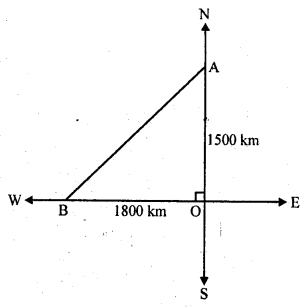Speed of the first plane = 1000 km/hr
Distance travelled in 1$$\frac { 1 }{ 2 }$$ hour due north = 1000 x $$\frac { 3 }{ 2 }$$ = 1500 km
Speed of the second plane = 1200 km/hr
Distance travelled in 1$$\frac { 1 }{ 2 }$$ hours due west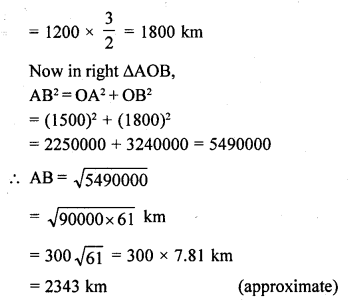Hope given RD Sharma Class 10 Solutions Chapter 7 Triangles Ex 7.7 are helpful to complete your math homework.

If you have any doubts, please comment below. Learn Insta try to provide online math tutoring for you.